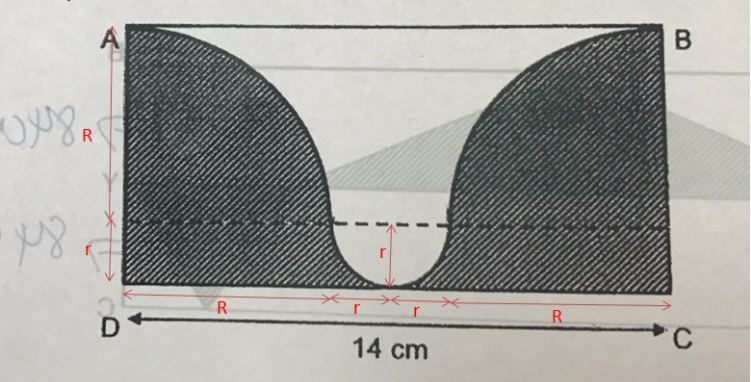# QuestionCD = R + r + r + R = 14 cm

2R + 2r = 14

2(R + r) = 14

R + r = 7 cm

AD = BC = (R + r) = 7 cm

Perimeter of big semicircle (2 × quarter circle)

½ × 2πR = πR

Perimeter of small semicircle → ½ × 2πr = πr

Total Perimeter → 7 + 14 + 7 + πR + πr = 28 + π(R + r) = 28 + 22/7 × 7 = 50 cm

0 Replies 0 Likes ✔Accepted Answer

Drawing a perpendicular line through the centre of the semicircle, AD = BC = 7 cm.
total perimeter of the shaded part ——- 7 + 14 + 7 + (1/2) x (22/7) x 14 = 50

Ans : 50 cm.